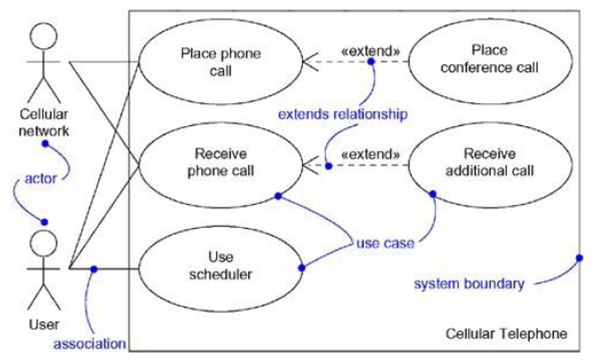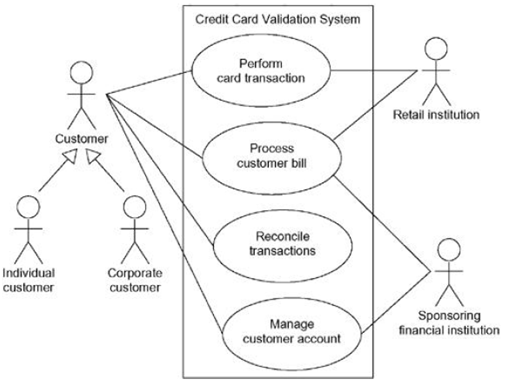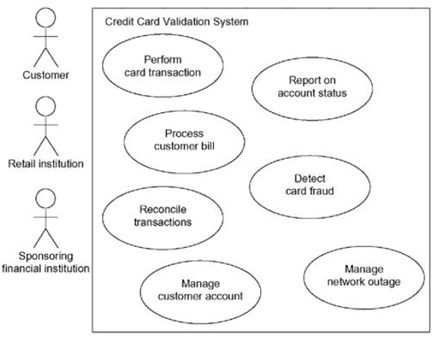UML Tutorial for Beginners
Blog containing resources for uml like lecture notes, lecture videos, lab manual, uml diagrams, objective bits, important questions and more.

11/08/2013 Categories: Behavioral Modeling.

Use case diagrams are one of the five behavioral diagrams for modeling the dynamic aspects of the system. A use case diagram specifies what the system does (behavior) for the actors.

Use case diagram plays a key role in modeling the behavior of a system or subsystem or a class. Each use case diagram consists of a set of use cases, actors and their relationships.

## Common Properties

Use case diagrams shares some common properties with the rest of the UML diagrams like a name which identifies a use case diagram and the graphical content which is a projection into a model.

## Content

Use case diagrams commonly contain:

• Use cases
• Actors
• Dependency, generalization and association relationships

Like all other diagrams, use case diagrams may contain notes and constraints. They may also contain packages or subsystems, which are used to group elements of your model into larger chunks.

## Common Uses

When we model the static use case view of a system, we apply use case diagrams in one of the two ways:

• To model the context of a system
• To model the requirements of a system

Example:## Common Modeling Techniques

### Modeling the context of a system

All the things (actors) that live outside the system and interact with the system constitute the system’s context. This context defines the environment in which the system lives.

To model the context of a system:

1. Identify the actors that surround the system.
2. Organize actors that are similar to one another in a generalization-specialization hierarchy.
3. For better semantics, provide a stereotype for each actor.
4. Create a use case diagram with these actors and specify the paths of communication for each actor to the system’s uses cases using association relationships.### Modeling the requirements of a system

A system’s functional requirements can be expressed as use cases, and the UML’s use case diagrams are essential for managing these requirements.

To model the requirements of a system:

1. Establish the context of the system by identifying the actors that surround (use) the system.
2. For each actor, identify the behavior that the actor expects from the system or requires the system to provide.
3. Name these common behaviors as use cases.
4. Use include and extend relationships to factor common behavior and optional behavior.
5. Model these use cases, actors and their relationships in a use case diagram.
6. Use notes to provide details about the use cases and other non-functional requirements.### Forward and reverse engineering

To forward engineer a use case diagram:

1. For each use case, identify the flow of events and its exceptional flow of events.
2. Generate a test script for each flow, using the flow’s preconditions as the test’s initial state and its post conditions as its success criteria.
3. As necessary, simulate test runs to represent each actor that interacts with the use case.
4. Use tools to run these tests each time you release the element to which the use case diagram applies.

To reverse engineer a use case diagram:

1. Identify each actor that interacts with the system.
2. For each actor, consider how the actor interacts with the system.
3. Trace the flow of events in the executable system relative to each actor.
4. Cluster (group) related flows by declaring a corresponding use case. Use include and extend relationships as necessary.
5. Model these actors and use cases in a use case diagram, and establish their relationships.

### Popular Posts:

Note: Do you have a question on this article or have a suggestion to make this article better? You can ask or suggest us by filling in the below form. After commenting, your comment will be held for moderation and will be published in 24-48 hrs.